2022: SklogWiki celebrates 15 years on-line

# Hard hyperspheres

(diff) ← Older revision | Latest revision (diff) | Newer revision → (diff)

Hard hyperspheres are hard spheres in more than three dimensions (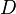$D$).

## Volume

The volume of an n-sphere of unit diameter is given by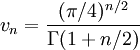$v_n=\frac{(\pi / 4)^{n/2}}{\Gamma(1+ n/2)}$

where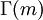$\Gamma(m)$ is the Gamma function.

## Liquid-solid transition

The liquid-solid transition for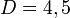$D=4, 5$ and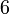$6$ .

## Virial coefficients

Hard sphere: virial coefficients for odd dimensions 

## Percus–Yevick equation

The Percus Yevick equation